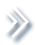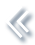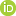####ArchivesReview Articles

## Multivariate small area estimation under nonignorable nonresponse*

Danny Pfeffermann ,

National Statistician and CBS Director, Jerusalem, Israel; Department of Statistics, Hebrew University, Jerusalem, Israel; Southampton Statistical Sciences Research Institute (S3RI), University of Southampton, Southampton, UKMichael Sverchkov

Bureau of Labor Statistics, Washington, DC, USAsverchkov.michael@bls.gov

Pages 213-223 | Received 01 Jan. 2019, Accepted 02 Oct. 2019, Published online: 22 Oct. 2019,
• Abstract
• References
• Citations

ABSTRACT

We consider multivariate small area estimation under nonignorable, not missing at random (NMAR) nonresponse. We assume a response model that accounts for the different patterns of the observed outcomes, (which values are observed and which ones are missing), and estimate the response probabilities by application of the Missing Information Principle (MIP). By this principle, we first derive the likelihood score equations for the case where the missing outcomes are actually observed, and then integrate out the unobserved outcomes from the score equations with respect to the distribution holding for the missing data. The latter distribution is defined by the distribution fitted to the observed data for the respondents and the response model. The integrated score equations are then solved with respect to the unknown parameters indexing the response model. Once the response probabilities have been estimated, we impute the missing outcomes from their appropriate distribution, yielding a complete data set with no missing values, which is used for predicting the target area means. A parametric bootstrap procedure is developed for assessing the mean squared errors (MSE) of the resulting predictors. We illustrate the approach by a small simulation study.

## References

1. Cepillini, R., Siniscialco, M., & Smith, C. A. B. (1955). The estimation of gene frequencies in a random mating population. Annals of Human Genetics20, 97–115. doi: 10.1111/j.1469-1809.1955.tb01360.x [Crossref][Web of Science ®], [Google Scholar]
2. Little, R. J. A. (1982). Models for nonresponse in sample surveys. Journal of the American Statistical Association77, 237–250. doi: 10.1080/01621459.1982.10477792 [Taylor & Francis Online][Web of Science ®], [Google Scholar]
3. Little, R. J. A., & Rubin, D. B. (2002). Statistical analysis with missing data (2nd ed.). New York: Wiley. [Crossref], [Google Scholar]
4. Orchard, T., & Woodbury, M. A. (1972). A missing information principle: Theory and application. Proceedings of the 6th Berkeley Symposium on Mathematical Statistics and Probability1, 697–715. [Google Scholar]
5. Pfeffermann, D., & Sikov, N. (2011). Imputation and estimation under nonignorable nonresponse in household surveys with missing covariate information. Journal of Official Statistics27, 181–209. [Web of Science ®], [Google Scholar]
6. Pfeffermann, D., & Sverchkov, M. (2007). Small-area estimation under informative probability sampling of areas and within selected areas. Journal of the American Statistical Association102, 1427–1439. doi: 10.1198/016214507000001094 [Taylor & Francis Online][Web of Science ®], [Google Scholar]
7. Riddles, K. M., Kim, J. K., & Im, J. (2016). A propensity-score adjustment method for nonignorable nonresponse. Journal of Survey Statistics and Methodology4, 215–245. doi: 10.1093/jssam/smv047 [Crossref], [Google Scholar]
8. Rubin, D. B. (1976). Inference and missing data. Biometrika63, 581–590. doi: 10.1093/biomet/63.3.581 [Crossref][Web of Science ®], [Google Scholar]
9. Sverchkov, M. (2008). A new approach to estimation of response probabilities when missing data are not missing at random. Joint statistical Meetings. Proceedings of the section on survey research methods (pp. 867–874). [Google Scholar]
10. Sverchkov, M., & Pfeffermann, D. (2004). Prediction of finite population totals based on the sample distribution. Survey Methodology30, 79–92. [Google Scholar]
11. Sverchkov, M., & Pfeffermann, D. (2018). Small area estimation under informative sampling and not missing at random nonresponse. Journal of the Royal Statistical Society JRSS-SA181, 981–1008. doi: 10.1111/rssa.12362 [Crossref][Web of Science ®], [Google Scholar]